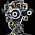## 2/29/2016

### SVD function built-in matlab convert to c++ code using matlab coder app.

http://study.marearts.com/2016/02/matlab-coder-simple-test-and-practical.html

Well, I have a interesting question.
It is also used to convert the MATLAB built-in functions to c ++ code?
example.. SVD function.

SVD(Singular Value Decomposition) is very useful function for solving linear algebra problem.
But it is difficult to find the source only pure c code, Often including a linear algebra as big library.

So, this article aims to convert SVD built in matlab function to c code and use the converted c code in Visual studio.

First, a start by calculating a fixed value of 4x2  matrix.

This is my example matrix and result.
This is an example in matlab help document.

First, let's look matlab code, it is very simple.
MySVD.m
///
```function [U, S, V] = MySVD(X)

[U, S, V]= svd(X);
```
///

testMySVD.m
///
```X=[ 1 2
3 4
5 6
7 8
];

[U, S, V] = MySVD(X);

U
S
V
U*S*V'
```
///

result

Now, let's convert MySVD.m to c code using matlab corder.

However, options for the input X is set to 4x2 double.

next,
An example using the code of MySVD c code in Visual Studio.
The result of visual studio is same with matlab.

MySVD C code is uploaded in github.

///
```#include < iostream>
#include "MySVD.h"
using namespace std;

void main()
{
double X[]={1, 3, 5, 7, 2, 4, 6, 8};
double U; //4x4
double S; //4x2
double V; //2x2

//extern void MySVD(const real_T X, real_T U, real_T S, real_T V);

MySVD(X, U, S, V);

cout << "U out" << endl;
for(int i=0; i< 4; ++i) //row
{
for(int j=0; j< 4; ++j) //col
cout << U[i+j*4] << " ";
cout << endl;
}
cout << endl;

cout << "S out" << endl;
for(int i=0; i< 4; ++i) //row
{
for(int j=0; j< 2; ++j) //col
{
cout << S[i+j*4] << " ";

}
cout << endl;
}
cout << endl;

cout << "V out" << endl;
for(int i=0; i< 2; ++i) //row
{
for(int j=0; j< 2; ++j) //col
{
cout << V[i+j*2] << " ";

}
cout << endl;
}
cout << endl;

}
```
///

The sign is a little different
But value of U* S * V 'is as correct.
And sign is irrelevant since the opposite direction to the perpendicular direction.

Next let's study the use of nxm svd

First, let's create the code from matlab data type of NxN

:inf x :inf is nxn.
After code build, see the this c++ code

///
```#include < iostream>
#include "MySVD.h"
#include "MySVD_emxAPI.h" //for emxArray_real_T
using namespace std;

void main()
{
double X[] = { 1, 3, 5, 7, 2, 4, 6, 8, 1, 2, 3, 4};
double U; //4x4
double S; //4x3
double V; //3x3

emxArray_real_T *inputX, *outputV, *outputS, *outputU;
inputX = emxCreateWrapper_real_T(&(X), 4, 3);
outputU = emxCreateWrapper_real_T(&(U), 4, 4);
outputS = emxCreateWrapper_real_T(&(S), 4, 3);
outputV = emxCreateWrapper_real_T(&(V), 3, 3);

//extern void MySVD(const emxArray_real_T *X, emxArray_real_T *U, emxArray_real_T *S, emxArray_real_T *V);
MySVD(inputX, outputU, outputS, outputV);

cout << "U out" << endl;
for (int i = 0; i< 4; ++i) //row
{
for (int j = 0; j< 4; ++j) //col
cout << U[i + j * 4] << " ";
cout << endl;
}
cout << endl;

cout << "S out" << endl;
for (int i = 0; i< 4; ++i) //row
{
for (int j = 0; j< 3; ++j) //col
{
cout << S[i + j * 4] << " ";

}
cout << endl;
}
cout << endl;

cout << "V out" << endl;
for (int i = 0; i< 3; ++i) //row
{
for (int j = 0; j< 3; ++j) //col
{
cout << V[i + j * 3] << " ";

}
cout << endl;
}
cout << endl;

}
```
///

The input of dynamic size is set by "emxArray_real_T" type.
for example const emxArray_real_T *X

transfer double value to emxArray_real_T, we can use "emxCreateWrapper_real_T" function.
For this function, don't forget include "MySVD_emxAPI.h" or "xxxx_emxAPI.h".

You code like this.
inputX = emxCreateWrapper_real_T(&(X), 4, 2);
or
outputU = emxCreateWrapper_real_T(&(U), 4, 4);

double address is connected with emxArray_real_T.

Please check I have posted the source code in Github.

SVD 4x2 test
https://github.com/MareArts/Matlab-corder-test-SVD/tree/master/MySVD_test_4x2input
SVD 4xn test
https://github.com/MareArts/Matlab-corder-test-SVD/tree/master/MySVD_test_4xninput
SVD nx2 test
https://github.com/MareArts/Matlab-corder-test-SVD/tree/master/MySVD_test_nx2input
SVD 4x4 test
https://github.com/MareArts/Matlab-corder-test-SVD/tree/master/MySVD_test_NxN_input

1.Anonymous2/3/16 15:29

A simple example that explain the use of Matlab coder and mex file is presented here:

1.Thank you. but what is different mex and c/c++ static library option?
isn't it mex purpose to using in matlab?

2.Anonymous3/3/16 06:49

The c/c++ library contains all needed c files and mex gives the 'NounFunction_mex.c' file that is used to test the c code in Matlab

1.I see.
Thank you.

3.Hi! This is very useful but would you please explain why the sign is difference? Thank you.